## Saturday 11 July 2015

### VBA Code Indenter and Highlighting VBA Code

This week I was looking at my VBA code indenter addin.

Attention: The VBA code indenter has recently been replaced by AET VBE Tools. Download them here.

First thing I did was make some improvements to the menu code. Some people have mentioned that they have trouble getting the menu to show, and I think I found the reason and fixed it.

I also added an option to indent single procedures. Just place your cursor in the procedure you want to indent, and select the appropriate option.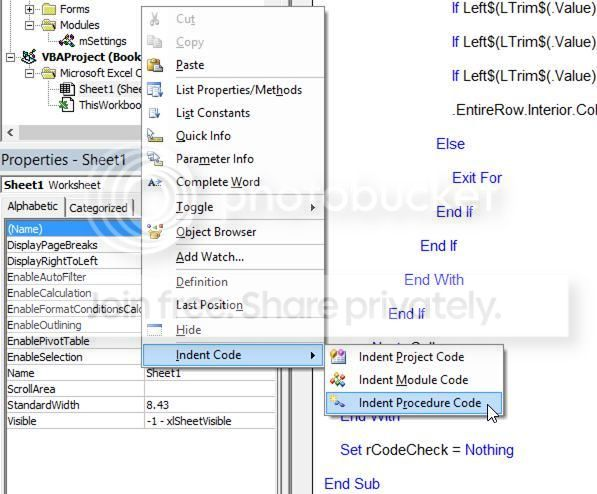I don't know how useful this is (why not just indent the whole module?), but I guess there are legitimate reasons. And I also did it because I wanted to see if I could! A productive learning experience in learning more VBE related code to be sure. To those interested, the project is password protected, but use "password" to unlock it and have a look at the code if you want.

Now regarding the Visual Basic Editor itself. You can make various changes to make your code more visible on the Editor Format tab on the Options dialog box (click Tools, Option on the top menu of the Visual Basic Editor). The forecolour of your Comment Text is probably set to green by default. Another change I like is to make Keywords Text blue. Keywords include Sub, Function, If, Then, etc.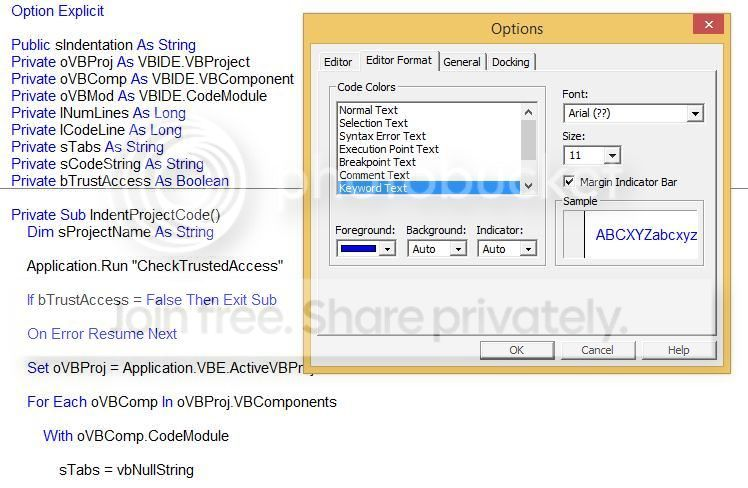Another thing I'd really like to do though is to dynamically highlight "matching" text, and everything in between. What do I mean by this? Pictures are probably an easy way to explain.

Last time I looked at indenting VBA code in cells instead of the Visual Basic Editor.

As far as I know, you can't change the colour of VBA code in the VBE, apart from Tools, Options, Editor Format mentioned above. (Am I wrong? Let me know!).

But code in a worksheet is a different situation. You can use a Worksheet_SelectionChange event to do all kinds of things!

Before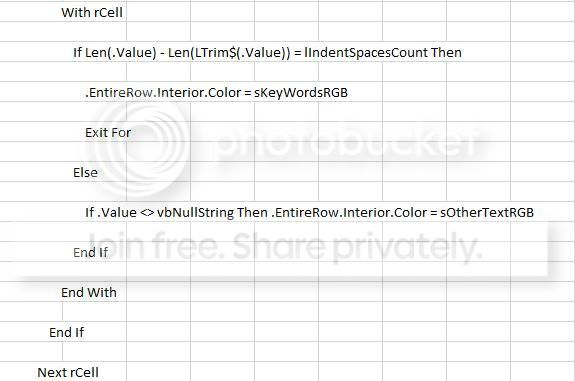After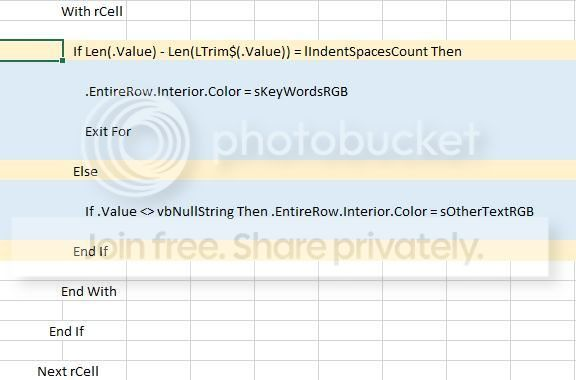Useful? Maybe, if you have lots of code and it's driving you crazy to see which code matches what. You might have lots of nested code, or code off screen. Yes, we can break code down into smaller subroutines or functions, but that's a job in itself, and how about if the code is not ours to change? I think highlighting code like this is a possibly useful curiosity in any case.

Here's the highlight code. In the VBE, paste it into the Sheet module that corresponds with the sheet where your code is to be highlighted.
```Private Sub Worksheet_SelectionChange(ByVal Target As Range)
Dim rCodeCheck As Range
Dim rCell As  Range
Dim lIndentSpacesCount As String
Dim sFirstWord As String
Dim sKeyWordsRGB As String
Dim sOtherTextRGB As String

Cells.Interior.ColorIndex = xlNone

sKeyWordsRGB = RGB(255, 242, 204) 'Change to suit

sOtherTextRGB = RGB(221, 235, 247) 'Change to suit

With Target

If .Column <> 1 Then Exit Sub

If .Cells.Count > 1 Then Exit Sub

If .Value = vbNullString Then Exit Sub

Set rCodeCheck = Range(Cells(1, 1), Cells(Rows.Count, 1).End(xlUp))

If Intersect(Target, rCodeCheck) Is Nothing Then

Set rCodeCheck = Nothing

Exit Sub

End If

lIndentSpacesCount = Len(.Value) - Len(LTrim\$(.Value))

If InStr(LTrim\$(.Value), Chr(32)) > 0 Then

sFirstWord = Left\$(LTrim\$(.Value), InStr(LTrim\$(.Value), Chr(32)) - 1)

Else

sFirstWord = vbNullString

End If

Select Case sFirstWord

Case "Select", "For", "Do", "With"

.EntireRow.Interior.Color = sKeyWordsRGB

For Each rCell In rCodeCheck.Cells

If rCell.Row > .Row Then

With rCell

If Len(.Value) - Len(LTrim\$(.Value)) = lIndentSpacesCount Then

.EntireRow.Interior.Color = sKeyWordsRGB

Exit For

Else

If .Value <> vbNullString Then .EntireRow.Interior.Color = sOtherTextRGB

End If

End With

End If

Next rCell

Case "If", "#If"

If Right(.Value, 4) = "Then" Then

.EntireRow.Interior.Color = sKeyWordsRGB

For Each rCell In rCodeCheck.Cells

If rCell.Row > .Row Then

With rCell

If Len(.Value) - Len(LTrim\$(.Value)) = lIndentSpacesCount Then

.EntireRow.Interior.Color = sKeyWordsRGB

If Left\$(LTrim\$(.Value), 6) = "End If" Then Exit For

If Left\$(LTrim\$(.Value), 7) = "#End If" Then Exit For

Else

If .Value <> vbNullString Then .EntireRow.Interior.Color = sOtherTextRGB

End If

End With

End If

Next rCell

Else

.EntireRow.Interior.Color = sOtherTextRGB

For Each rCell In rCodeCheck.Cells

If rCell.Row > .Row Then

With rCell

If .Value <> vbNullString Then

If Len(.Value) - Len(LTrim\$(.Value)) = lIndentSpacesCount Then

If Left\$(LTrim\$(.Value), 6) = "Select" Then Exit For

If Left\$(LTrim\$(.Value), 3) = "For" Then Exit For

If Left\$(LTrim\$(.Value), 2) = "Do" Then Exit For

If Left\$(LTrim\$(.Value), 4) = "Loop" Then Exit For

If Left\$(LTrim\$(.Value), 4) = "With" Then Exit For

If Left\$(LTrim\$(.Value), 2) = "If" And Right\$(.Value, 4) = "Then" Then Exit For

If Left\$(LTrim\$(.Value), 3) = "#If" And Right\$(.Value, 4) = "Then" Then Exit For

.EntireRow.Interior.Color = sOtherTextRGB

Else

Exit For

End If

End If

End With

End If

Next rCell

End If

Case "End", "#End", "Loop"

'Do nothing

Case Else

.EntireRow.Interior.Color = sOtherTextRGB

For Each rCell In rCodeCheck.Cells

If rCell.Row > .Row Then

With rCell

If .Value <> vbNullString Then

If Len(rCell.Value) - Len(LTrim\$(.Value)) = lIndentSpacesCount Then

If Left\$(LTrim\$(.Value), 6) = "Select" Then Exit For

If Left\$(LTrim\$(.Value), 3) = "For" Then Exit For

If Left\$(LTrim\$(.Value), 2) = "Do" Then Exit For

If Left\$(LTrim\$(.Value), 4) = "Loop" Then Exit For

If Left\$(LTrim\$(.Value), 4) = "With" Then Exit For

If Left\$(LTrim\$(.Value), 2) = "If" And Right\$(.Value, 4) = "Then" Then Exit For

If Left\$(LTrim\$(.Value), 3) = "#If" And Right\$(.Value, 4) = "Then" Then Exit For

.EntireRow.Interior.Color = sOtherTextRGB

Else

Exit For

End If

End If

End With

End If

Next rCell

End Select

End With

Set rCodeCheck = Nothing

End Sub
```

Now I have to say something. The Visual Basic Editor has seen little change over a long period of time. It certainly doesn't have the rich interface functionality of other code editors. And trying to find code samples to manipulate the interface is not an easy task.

Will this change? There's been many changes to the front end of Excel and other Office applications. The Visual Basic Editor is wanting, at least as the interface is concerned.

Microsoft, I'm sure there's many programmers besides myself who would like to see improvements. Our numbers may pale in comparison compared to ordinary users, but Office is not cheap. I would definitely like to see something better, coming soon.

Opinions? I'd like to see your feedback in the comments. (and like I wrote above, I'd also like to see a way of highlighting code within the VBE if it can be done.)

1.All these years, hell decades, and I never noticed I could highlight keywords?!? Brings my IDE up to the mid-2000s.

The generally-accepted wisdom is that the VBE will never be updated. I can't think why that would change, except to support a tool that must be used by hundreds of thousands, if not millions, of Office users at some point or other.

1.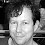I wasn't aware either Doug. I had played around with other settings before, but only came across this functionality last week :-)

Yeah, about the VBE, I'm not optimistic but who knows. It would certainly be good PR if Microsoft did improve a few things just to let us developers know we are not forgotten (I'm guessing you've seen Steve Ballmers's "Developers" video on YouTube!).

Anyway, I'm going to dig around and do some research. Maybe I can come up with something.